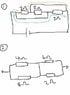# Electric Circuit - Equivalent Resistance

• leslielau

## Homework Statement

For 1 and 2 : Find the equivalent resistance for the resistor network provided

## Homework Equations

For resistor in series : Req = R$_{1}$+R$_{2}$+...R$_{n}$

For resistors in parallel : Req = (1/R$_{1}$+1/R$_{2}$+... 1/R$_{n}$)$^{-1}$

## The Attempt at a Solution

For question 1 :

I have considered the two paths shown :

1st path (blue) : total resistance = 2 ohm
2nd path (orange) : total resistance = 2 ohm

so since these two paths are in parallel , Req = 1 ohm

But answer provided = 2/3 ohm

For Question 2 :

Since no current passes through the middle wire, upper path = 10 ohm
lower path = 10 ohm

So, total resistance = 5 ohm

However , the answer used the method of (1/4+1/8)^-1 + (1/2+1/6)^-1 = 4.17 ohm

But I don't understand this part, if there is current flowing in the middle wire, there would be one current flowing down and one current flowing up. Won't they cancel out each other? Thanks !

#### Attachments

•Resistor.jpg
16.8 KB · Views: 359

## Homework Statement

For 1 and 2 : Find the equivalent resistance for the resistor network provided

## Homework Equations

For resistor in series : Req = R$_{1}$+R$_{2}$+...R$_{n}$

For resistors in parallel : Req = (1/R$_{1}$+1/R$_{2}$+... 1/R$_{n}$)$^{-1}$

## The Attempt at a Solution

For question 1 :

I have considered the two paths shown :

1st path (blue) : total resistance = 2 ohm
2nd path (orange) : total resistance = 2 ohm

so since these two paths are in parallel , Req = 1 ohm

But answer provided = 2/3 ohm

For Question 2 :

Since no current passes through the middle wire, upper path = 10 ohm
lower path = 10 ohm

So, total resistance = 5 ohm

However , the answer used the method of (1/4+1/8)^-1 + (1/2+1/6)^-1 = 4.17 ohm

But I don't understand this part, if there is current flowing in the middle wire, there would be one current flowing down and one current flowing up. Won't they cancel out each other? Thanks !

For question 1 there is a third path you have not considered.

I have thought of two : One through the middle through all 3 resistors, and another one , turning left between middle and rightmost resistor to pass through the middle resistor.

Which one is correct?

## Homework Statement

For 1 and 2 : Find the equivalent resistance for the resistor network provided

## Homework Equations

For resistor in series : Req = R$_{1}$+R$_{2}$+...R$_{n}$

For resistors in parallel : Req = (1/R$_{1}$+1/R$_{2}$+... 1/R$_{n}$)$^{-1}$

## The Attempt at a Solution

For question 1 :

I have considered the two paths shown :

1st path (blue) : total resistance = 2 ohm
2nd path (orange) : total resistance = 2 ohm

so since these two paths are in parallel , Req = 1 ohm

But answer provided = 2/3 ohm

For Question 2 :

Since no current passes through the middle wire, upper path = 10 ohm
lower path = 10 ohm

So, total resistance = 5 ohm

However , the answer used the method of (1/4+1/8)^-1 + (1/2+1/6)^-1 = 4.17 ohm

But I don't understand this part, if there is current flowing in the middle wire, there would be one current flowing down and one current flowing up. Won't they cancel out each other? Thanks !

## The Attempt at a Solution

For the second part, there may well be current flowing through the middle wire - but since we consider a wire to have zero resistance, the potential at each end will be the same.
re-draw the circuit with the centre wire much, much shorter [like zero length] and see what the circuit looks like. you should then see where their answer comes from.

EDIT: Sorry but i don't know how to load pictures

I have thought of two : One through the middle through all 3 resistors, and another one , turning left between middle and rightmost resistor to pass through the middle resistor.

Which one is correct?

The second - as it offers another path back to the battery having passed through only one of the resistors - the same as the two paths you identified at first.

I have thought of two : One through the middle through all 3 resistors, and another one , turning left between middle and rightmost resistor to pass through the middle resistor.

Which one is correct?

Try this: use a high-lite pen to high-lite the wire from the positive terminal of the battery. continue shading as far as you can along any wires without passing through a resistor, then have a look at what you have shaded.

Preferably with a different colour, start at the negative terminal and shade back along wires - but again don't go through any resistor.

What do you notice?

EDIT: It is 2:30 am here, I am off to bed!